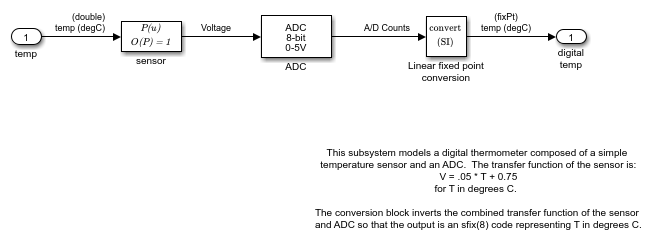Documentation

Model a Digital Thermometer Using the Polynomial Block

This example shows how the ex_sldemo_boiler model uses the Polynomial block.

In the Boiler Plant model/digital thermometer subsystem, the Polynomial Block models a first-order polynomial using the coefficients [0.05 0.75]